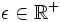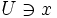Equicontinuous family of functions

Let$F$ be a family of functions from a topological space$X$ to a metric space$Y$. Then we say that the functions are equicontinuous if given any$x \in X$, and any$\epsilon \in \R^+$, there is an open set$U \ni x$ such that$d(f(x),f(x')) < \epsilon \forall x\ \in U, f \in F$.